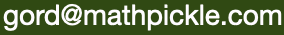Select Page

#### Uncracked 114

##### (Diophantus of Alexandria, c. 215-290)

Students working with exponents should be asked to find which values of n from 0-100 are possible by summing the cubes of three positive integers: a^3 + b^3 + c^3 = n.

Next, ask if the cubes can be negative. This is unsolved as you can see in the video, but do not tell your students this till the end of class! This video might be the best way to end the class – or to start the next class.

http://mathoverflow.net/ is a recommended resource for math entusiasts. It shows some of the history of this problem for discoveries n≤100:

(1960s)

87=4271³–4126³–1972³
96=−15250³+13139³+10853³
91=83538³–67134³–65453³
80=−112969³+103532³+69241³

(1990s)

39=−159380³+134476³+117367³
75–435203231³+435203083³+4381159³
84=41639611³–41531726³–8241191³

(2000s)

30=2220422932³–2218888517³–283059965³
52=−61922712865³+23961292454³+60702901317³
74=−284650292555885³+66229832190556³+283450105697727³

For n≤1000, the problem is still open only for 33, 42, 114, 165, 390, 579, 627, 633, 732, 795, 906, 921, and 975

This problem also lends itself to algebraic exploration, but only for elite classes. As well as the algebraic exploration seen in the video check out Euler-Binet solutions here.

________________________

Hi Gord,

Just thought you should know significant progress has been made; the website currently reads “For n≤1000, the problem is still open only for 33, 42, 114, 165, 390, 579, 627, 633, 732, 795, 906, 921, and 975”

In 2019, both 33 and 42 have been solved (the former by Andrew Booker, and the latter by Andrew Booker and Andrew Sutherland):
33 = (-8 778 405 442 862 239)^3 + (-2 736 111 468 807 040)^3 + (8 866 128 975 287 528)^3
42 = (-80 538 738 812 075 974)^3 + (80 435 758 145 817 515)^3 + (12 602 123 297 335 631)^3

As far as I’m aware, the other 11 numbers on your list remain the only unsolved cases below 1000.

Cheers,

Vince

Monday, April 4, 2022

________________________

I was wrong – there are other solutions that Booker found in 2019:

165 = (-385 495 523 231 271 884)^3 + (383 344 975 542 639 445)^3 + (98 422 560 467 622 814)^3
795 = (-14 219 049 725 358 227)^3 + (2 337 348 783 323 923)^3 + (14 197 965 759 741 571)^3
906= (-74 924 259 395 610 397)^3 + (35 961 979 615 356 503)^3 + (72 054 821 089 679 353 378)^3

So the 8 remaining are:
114, 390, 579, 627, 633, 732, 921, and 975

Vince

Monday, April 4, 2022

________________________

I shared this email exchange with Vince because it is kind of typical of correspondence among mathematicians. Vince Chan is on faculty at the University of Calgary.

#### Standards for Mathematical Practice

MathPickle puzzle and game designs engage a wide spectrum of student abilities while targeting the following Standards for Mathematical Practice:

##### MP1 Toughen up!

Students develop grit and resiliency in the face of nasty, thorny problems. It is the most sought after skill for our students.

##### MP2 Think abstractly!

Students take problems and reformat them mathematically. This is helpful because mathematics lets them use powerful operations like addition.

##### MP3 Work together!

Students discuss their strategies to collaboratively solve a problem and identify missteps in a failed solution. MathPickle recommends pairing up students for all its puzzles.

##### MP4 Model reality!

Students create a model that mimics the real world. Discoveries made by manipulating the model often hint at something in the real world.

##### MP5 Know the tools.

Students master the tools at their fingertips - whether it's a pencil or an online app.

##### MP6 Be precise!

Students learn to communicate using precise terminology. MathPickle encourages students not only to use the precise terms of others, but to invent and rigorously define their own terms.

##### MP7 Be observant!

Students learn to identify patterns. This is one of the things that the human brain does very well. We sometimes even identify patterns that don't really exist 😉

##### MP8 Be lazy!?!

Students learn to seek for shortcuts. Why would you want to add the numbers one through a hundred if you can find an easier way to do it?

Please use MathPickle in your classrooms. If you have improvements to make, please contact me. I'll give you credit and kudos 😉 For a free poster of MathPickle's ideas on elementary math education go here.

## Gordon Hamilton

##### (MMath, PhD)